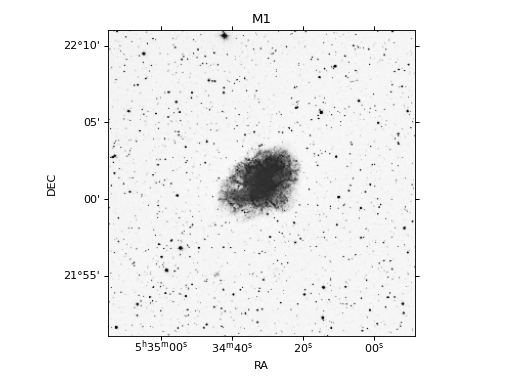# Plotting with Astroplan¶

`astroplan` currently has convenience functions for making three different types of plots: airmass vs time, parallactic angle vs time and sky charts. This plotting functionality in `astroplan` requires Matplotlib (although non- plotting functionality will work even without Matplotlib ). The use of additional plotting packages (like Seaborn) is not explicitly prevented, but may or may not actually work.

All `astroplan` plots return a `Axes` object (which by convention is assigned to the name `ax` in these tutorials). You can further manipulate the returned `ax` object, including using it as input for more `astroplan` plotting functions, or you can simply display/print the plot.

## Time Dependent Plots¶

Although all `astroplan` plots are time-dependent in some way, we label those that have a time-based axis as “time-dependent”.

`astroplan` currently has a few different types of “time-dependent” plots, for example `plot_airmass`, `plot_altitude` and `plot_parallactic`. These take, at minimum, `Observer`, `FixedTarget` and `Time` objects as input.

Airmass vs time plots are made the following way:

```>>> from astroplan.plots import plot_airmass

>>> plot_airmass(target, observer, time)
```

Parallactic angle vs time plots are made the following way:

```>>> from astroplan.plots import plot_airmass

>>> plot_parallactic(target, observer, time)
```

Below are general guidelines for working with time-dependent plots in `astroplan`. Examples use airmass but apply to parallactic angle as well.

### Making a quick plot¶

Any plot function in `astroplan` with a time-based axis will allow you to make a quick plot over a 24-hour period.

After constructing `Observer` and `FixedTarget` objects, construct a `Time` object with a single instance in time and issue the plotting command.

```>>> import matplotlib.pyplot as plt
>>> from astropy.time import Time
>>> from astroplan.plots import plot_airmass

>>> observe_time = Time('2000-06-15 23:30:00')

>>> plot_airmass(target, observer, observe_time)
>>> plt.show()
```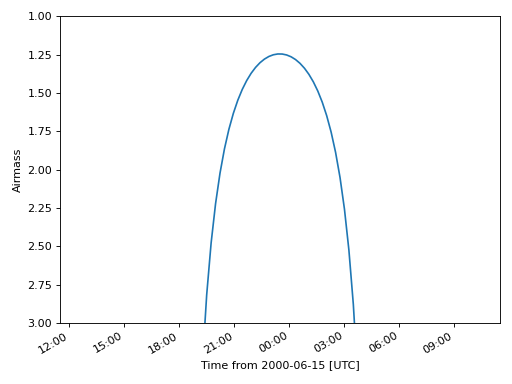As you can see, the 24-hour plot is centered on the time input. You can also use array `Time` objects for these quick plots–they just can’t contain more than one instance in time.

For example, these are acceptable time inputs:

```Time(['2000-06-15 23:30:00'])

[Time('2000-06-15 23:30:00')]
```

You can also add a second y-axis (on the right side) which shows the corresponding altitude of the targets: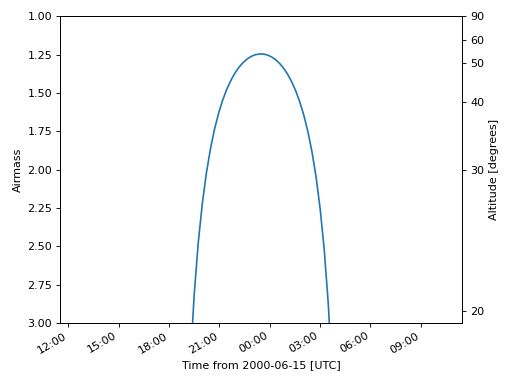You can make altitude the primary y-axis rather than airmass by using `plot_altitude`: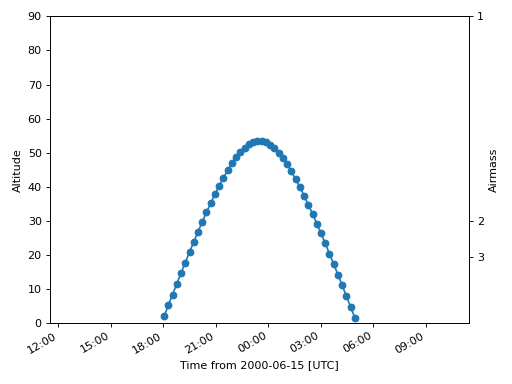### Specifying a time window¶

If you want to see airmass plotted over a window that is not 24 hours long or you want to control the precision of the plot, you must specify every time for which you want to see an airmass plotted. Therefore, an array `Time` object is necessary.

To quickly populate an `Time` object with many instances of time, use Numpy and `units`. See example below.

#### Centering the window at some time¶

To center your window at some instance in time:

```>>> import numpy as np
>>> import matplotlib.pyplot as plt
>>> import astropy.units as u
>>> from astropy.time import Time
>>> from astroplan.plots import plot_airmass

>>> observe_time = Time('2000-06-15 23:30:00')
>>> observe_time = observe_time + np.linspace(-5, 5, 55)*u.hour

>>> plot_airmass(target, observer, observe_time)
>>> plt.show()
```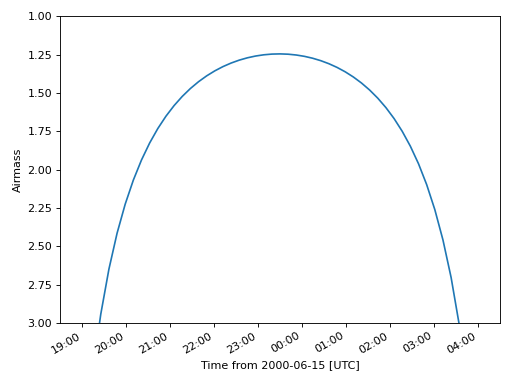#### Specify start and end times¶

If you know the start and end times of your observation run, you can use a `TimeDelta` object to create an array for time input:

```>>> import numpy as np
>>> import matplotlib.pyplot as plt
>>> from astropy.time import Time
>>> from astroplan.plots import plot_airmass

>>> start_time = Time('2000-06-15 20:00:00')
>>> end_time = Time('2000-06-16 04:00:00')
>>> delta_t = end_time - start_time
>>> observe_time = start_time + delta_t*np.linspace(0, 1, 75)

>>> plot_airmass(target, observer, observe_time)
>>> plt.show()
```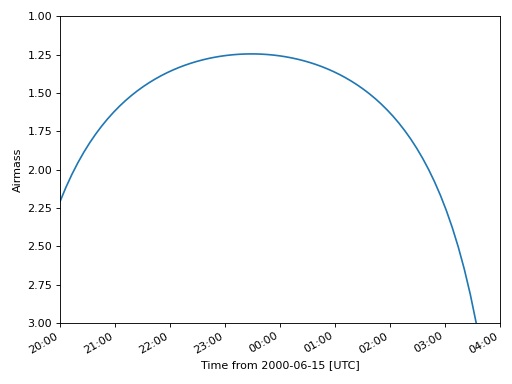### Plotting a quantity for multiple targets¶

If you want to plot airmass information for multiple targets, simply reissue the `plot_airmass` command, using a different `FixedTarget` object as input this time. Repeat until you have as many targets on the plot as you wish:

```>>> import numpy as np
>>> import matplotlib.pyplot as plt
>>> from astropy.time import Time
>>> from astroplan.plots import plot_airmass

>>> observe_time = Time('2000-06-30 23:30:00') + np.linspace(-7.0, 5.5, 50)*u.hour

>>> plot_airmass(target, observer, observe_time)
>>> plot_airmass(other_target, observer, observe_time)
>>> plot_airmass(third_target, observer, observe_time)

>>> plt.show()
```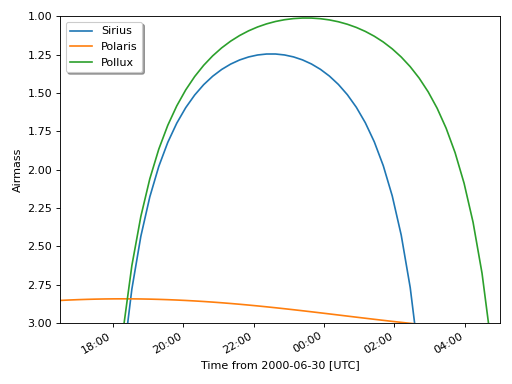When you’re ready to make a different plot, use `ax.cla()` to clear the current `Axes` object.

### Changing style options¶

The default line for time-dependent plots is solid and the default label (should you choose to display a legend) is the name contained in the `Target` object. You can change the linestyle, color, label and other plotting properties by setting the style_kwargs option.

```>>> import matplotlib.pyplot as plt
>>> from astroplan.plots import plot_airmass

>>> sirius_styles = {'linestyle': '--', 'color': 'r'}
>>> pollux_styles = {'color': 'g'}

>>> plot_airmass(target, observer, observe_time, style_kwargs=sirius_styles)
>>> plot_airmass(other_target, observer, observe_time, style_kwargs=pollux_styles)

>>> plt.show()
```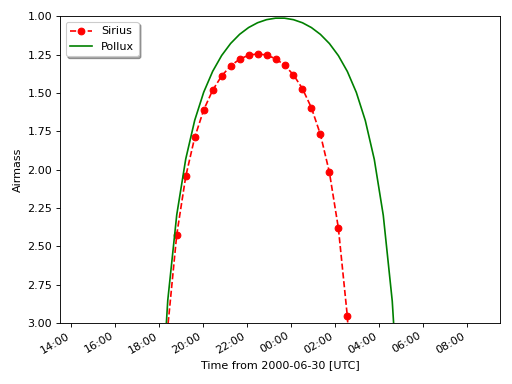See the Matplotlib documentation for information on plotting styles in line plots.

#### Dark Theme Plots¶

By default, `astroplan` uses the Astropy style sheet for Matplotlib to generate plots with more pleasing settings than provided for by the matplotlib defaults. When using `astroplan` at night, you may prefer to make plots with dark backgrounds, rather than the default white background, to preserve your night vision. To do so, you may use the `astroplan` dark style sheet to produce dark-themed plots by using the style_sheet keyword argument in any plotting function:

```>>> from astroplan.plots import dark_style_sheet, plot_airmass
>>> plot_airmass(target, observatory, time, style_sheet=dark_style_sheet)
```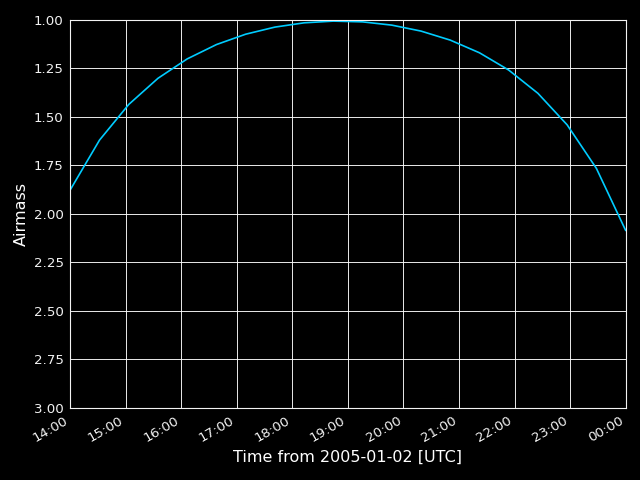You can also shade the background according to the darkness of the sky (light shading for 0 degree twilight, darker shading for -18 degree twilight) with the `brightness_shading` keyword, and display additional y-axis ticks on the right side of the axis with the altitudes in degrees using the `altitude_yaxis` keyword:

```>>> import matplotlib.pyplot as plt
>>> from astropy.time import Time
>>> from astroplan import FixedTarget, Observer
>>> from astroplan.plots import plot_airmass

>>> time = Time('2018-01-02 19:00')
>>> target = FixedTarget.from_name('HD 189733')
>>> apo = Observer.at_site('APO')
>>> plot_airmass(target, apo, time, brightness_shading=True, altitude_yaxis=True)
```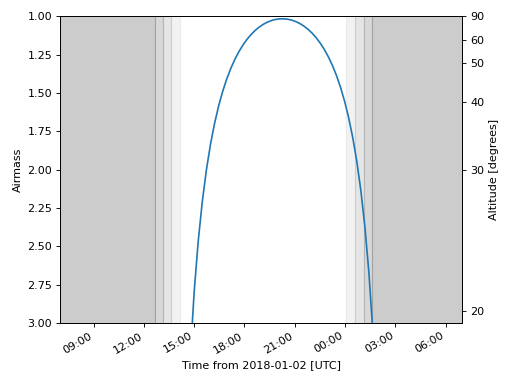## Sky Charts¶

Many users planning an observation run will want to see the positions of targets with respect to their local horizon, as well as the positions of familiar stars or other objects to act as guides.

`plot_sky` allows you to plot the positions of targets at a single instance or over some window of time. You make this plot the following way:

```>>> from astroplan.plots import plot_sky

>>> plot_sky(target, observer, time)
```

Note

`plot_sky` currently produces polar plots in altitude/azimuth coordinates only. Plots are centered on the observer’s zenith.

### Making a plot for one instance in time¶

After constructing your `Observer` and `FixedTarget` objects, construct a time input using an array of length 1.

That is, either an `Time` object with an array containing one time value (e.g., `Time(['2000-1-1'])`) or an array containing one scalar `Time` object (e.g., `[Time('2000-1-1')]`).

Let’s say that you created `FixedTarget` objects for Polaris, Altair, Vega and Deneb. To plot a map of the sky:

```>>> import matplotlib.pyplot as plt
>>> from astropy.time import Time
>>> from astroplan.plots import plot_sky

>>> observe_time = Time(['2000-03-15 15:30:00'])

>>> polaris_style = {color': 'k'}
>>> vega_style = {'color': 'g'}
>>> deneb_style = {'color': 'r'}

>>> plot_sky(polaris, observer, observe_time, style_kwargs=polaris_style)
>>> plot_sky(altair, observer, observe_time)
>>> plot_sky(vega, observer, observe_time, style_kwargs=vega_style)
>>> plot_sky(deneb, observer, observe_time, style_kwargs=deneb_style)

>>> plt.legend(loc='center left', bbox_to_anchor=(1.25, 0.5))
>>> plt.show()
```

Note

Since `plot_sky` uses `scatter` (which gives the same color to different plots made on the same set of axes), you have to specify the color for each target via a style dictionary if you don’t want all targets to have the same color.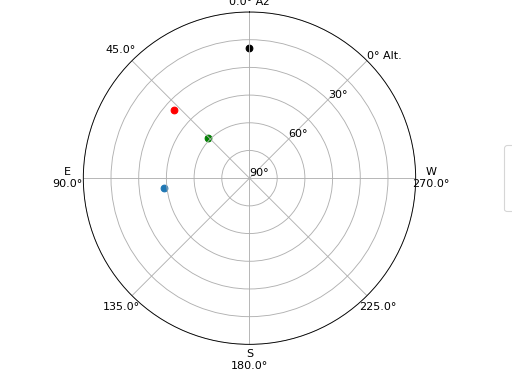### Showing movement over time¶

If you want to see how your targets move over time, you need to explicitly specify every instance in time.

Say I want to know how Altair moves in the sky over a 9-hour period:

```>>> import numpy as np
>>> import matplotlib.pyplot as plt
>>> import astropy.units as u
>>> from astroplan.plots import plot_sky

>>> observe_time = Time('2000-03-15 17:00:00')
>>> observe_time = observe_time + np.linspace(-4, 5, 10)*u.hour

>>> plot_sky(altair, observer, observe_time)

>>> plt.legend(loc='center left', bbox_to_anchor=(1.25, 0.5))
>>> plt.show()
```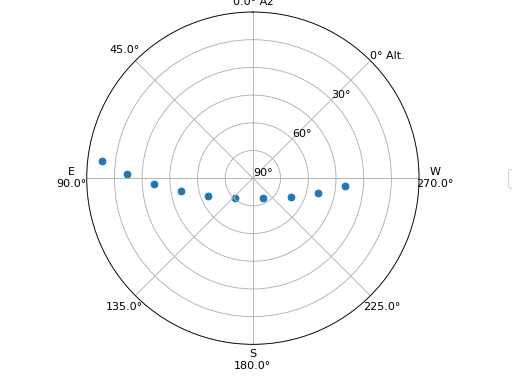For more examples on how to populate time objects, see `Time` documentation, or Specifying a time window.

Note

Note that in the case of an object being under the horizon (or having negative altitude) at any of the times in your time input, `plot_sky` will warn you. Your object(s) will not show up on the plot for those particular times, but any positions above the horizon will still be plotted as normal.

`astroplan` plots use Matplotlib defaults, so you can customize your plots in much the same way you tweak any Matplotlib plot.

#### Setting style options¶

The default marker for `plot_sky` is a circle and the default label (should you choose to display a legend) is the name contained in the `Target` object. You can change the marker, color, label and other plotting properties by setting the style_kwargs option, in the same way shown for the time-dependent plots.

One situation in which this is particularly useful is the plotting of guide positions, such as a few familiar stars or any body used in calibrating your telescope. You can also use this feature to set apart different types of targets (e.g., high-priority, candidates for observing run, etc.).

See the Matplotlib documentation for information on plotting styles in scatter plots.

#### Changing coordinate defaults¶

As seen in the above examples, the default position of North is at the top of the plot, and South at the bottom, with azimuth increasing counter-clockwise (CCW), putting East to the left, and West to the right.

You can’t change the position of North or South (either in the actual plotting of the data, or the labels), but you can “flip” East/West by changing the direction in which azimuth increases via the north_to_east_ccw option:

```>>> import matplotlib.pyplot as plt
>>> from astroplan.plots import plot_sky

>>> guide_style = {'marker': '*'}

>>> plot_sky(polaris, observer, observe_time, snorth_to_east_ccw=False, style_kwargs=guide_style)
>>> plot_sky(altair, observer, observe_time, north_to_east_ccw=False)

>>> plt.legend(loc='center left', bbox_to_anchor=(1.25, 0.5))
>>> plt.show()
```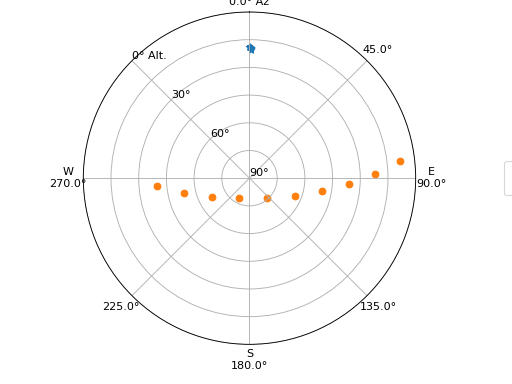Some observatories may need to offset or rotate the azimuth labels due to their particular telescope setup.

To do this, set az_label_offset equal to the number of degrees by which you wish to rotate the labels. By default, az_label_offset is set to 0 degrees. A positive offset is in the same direction as azimuth increase (see the north_to_east_ccw option):

```>>> import matplotlib.pyplot as plt
>>> from astroplan.plots import plot_sky

>>> guide_style = {'marker': '*'}

>>> plot_sky(polaris, observer, observe_time, style_kwargs=guide_style, az_label_offset=180.0*u.deg)
>>> plot_sky(altair, observer, observe_time, az_label_offset=180.0*u.deg)

>>> plt.legend(loc='center left', bbox_to_anchor=(1.25, 0.5))
>>> plt.show()
```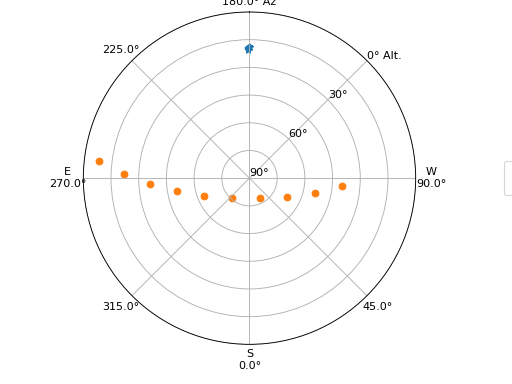Note

The az_label_offset option does not rotate the actual positions on the plot, but simply the theta grid labels (which are drawn regardless of gridline presence). Since labels are drawn with every call to `plot_sky`, we recommend you use the same az_label_offset argument for every target on the same plot.

It is not advised that most users change this option, as it may appear that your alt/az data does not coincide with the definition of altazimuth (local horizon) coordinate system.

You can turn off the grid lines by setting the grid option to False:

```>>> import matplotlib.pyplot as plt
>>> from astroplan.plots import plot_sky

>>> guide_style = {'marker': '*'}

>>> plot_sky(polaris, observer, observe_time, style_kwargs=guide_style, grid=False)
>>> plot_sky(altair, observer, observe_time, grid=False)

>>> plt.legend(loc='center left', bbox_to_anchor=(1.25, 0.5))
>>> plt.show()
```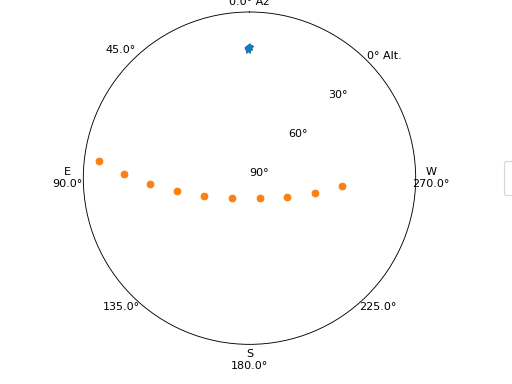Note

Since grids are redrawn with every call to `plot_sky`, you must set `grid=False` for every target in the same plot.

#### Other tweaks¶

You can easily change other plot attributes by acting on the returned `matplotlib.axes.Axes` object or via `matplotlib.pyplot` calls (e.g., `plt.figure`, `plt.rc`, etc.).

For instance, you can increase the size of your plot and its font:

```>>> # Set the figure size/font before you issue the plotting command.
>>> plt.figure(figsize=(8,6))
>>> plt.rc('font', size=14)

>>> plot_sky(polaris, observer, observe_time)
>>> plot_sky(altair, observer, observe_time)

>>> plt.legend(loc='center left', bbox_to_anchor=(1.25, 0.5))
>>> plt.show()

>>> # Change font size back to default once done plotting.
>>> plt.rc('font', size=12)
```

#### Miscellaneous¶

The easiest way to reuse the `Axes` object that is the base of your plots is to just let `astroplan`’s plotting functions take care of it in the background. You do, however, have the option of explicitly passing in a named axis, assuming that you have created the appropriate type for the particular plot you want.

We can explicitly give a name to the `Axes` object returned by `plot_sky` when plotting Polaris and reuse it to plot Altair:

```>>> my_ax = plot_sky(polaris, observer, observe_time, style_kwargs=guide_style)
>>> plot_sky(altair, observer, observe_time, my_ax)
```

We can also create a `Axes` object entirely outside of `plot_sky`, then pass it in:

```>>> my_ax = plt.gca(projection='polar')
>>> plot_sky(polaris, observer, observe_time, my_ax)
```

Passing in named `matplotlib.axes.Axes` objects comes in handy when you want to make multiple plots:

```>>> from astroplan.plots import plot_sky
>>> import matplotlib.pyplot as plt

>>> my_ax = plot_sky(polaris, observer, observe_time, style_kwargs=polaris_style)
>>> plot_sky(altair, observer, observe_time, my_ax, style_kwargs=altair_style)
>>> plt.legend(loc='center left', bbox_to_anchor=(1.3, 0.5))

>>> # Note that this plt.show (or another action, such as saving a figure) is critical in maintaining two separate plots.
>>> plt.show()

>>> other_ax = plot_sky(vega, observer, observe_time, style_kwargs=vega_style)
>>> plot_sky(deneb, observer, observe_time, other_ax, style_kwargs=deneb_style)
>>> plt.legend(loc='center left', bbox_to_anchor=(1.25, 0.5))
>>> plt.show()
```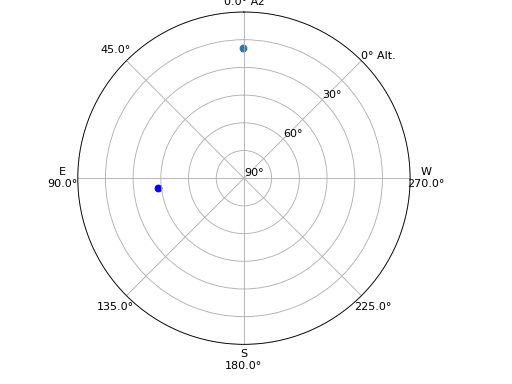(png, hires.png, pdf, svg)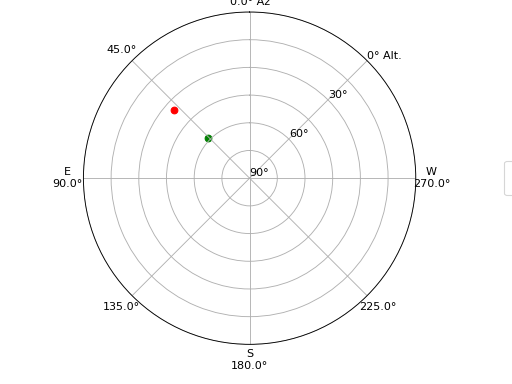## Finder Chart/Image¶

`astroplan` includes a function for generating quick finder images from Python, `plot_finder_image`, by querying for images from sky surveys centered on a `FixedTarget`. This function depends on astroquery (in addition to Matplotlib). In this example, we’ll quickly make a finder image centered on The Crab Nebula (M1):

```>>> from astroplan.plots import plot_finder_image
>>> from astroplan import FixedTarget
>>> import matplotlib.pyplot as plt

>>> messier1 = FixedTarget.from_name("M1")
>>> ax, hdu = plot_finder_image(messier1)
>>> plt.show()
```